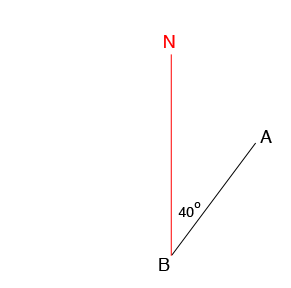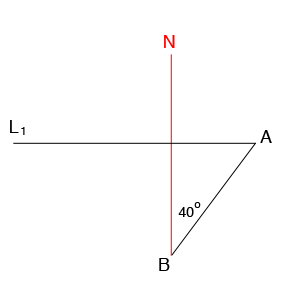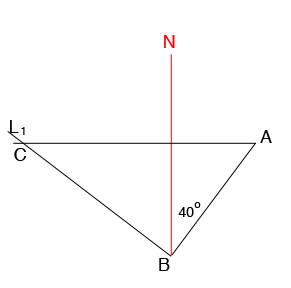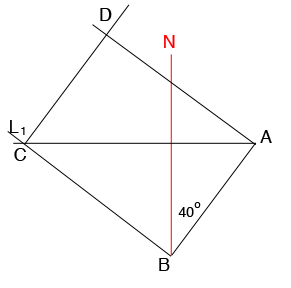SEARCH HOMEMath Central Quandaries & QueriesQuestion from Mubashir, a student: A,B,C,D are the four corners of a rectangular plot marked on level ground. Given that the bearing of B from A is 040 degrees and that the bearing of C from A is 090 degrees. Calculate the bearing of (a)B from C (b)A from C (c) D from CHo Mubashir,

I can help you with the diagram.

The bearing from $B$ to A is the angle between the line segment $BA$ and north, measuring clockwise.The bearing from $C$ to $A$ is 90 degrees and again the bearing is measured clockwise from north and thus $C$ must be on the east-west ray $L_1$ in the diagram below.From the diagram the measure of the angle $BAC$ is 60 degrees and hence $AC$ is not a side of the rectangle. Hence the angle measure of the angle $CBA$ is 90 degrees. Draw a line through $B$ which is perpendicular to $BA$ and this line must intersect the line $L_1$ at $C.$Finally draw a line through $C$ parallel to $BA$ and a line through $A$ parallel to $BC.$ These lines meet at $D$ the last corner of the rectangular plot.From the diagram you should now be able to determine the bearings required.

PennyMath Central is supported by the University of Regina and the Imperial Oil Foundation.